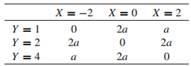# Let X and Y be two discrete random variables with joint probability mass function Let S and Z be…

Let X and Y be two discrete random variables with joint probability mass functionLet S and Z be two additional discrete random variables such that S = X + Y and Z = X − Y.

(a) Find a and the marginal probability mass function of X.

(b) Are X and Y independent?

(c) Construct the table of the joint probability mass function of S and Z.

(d) Are S and Z independent?